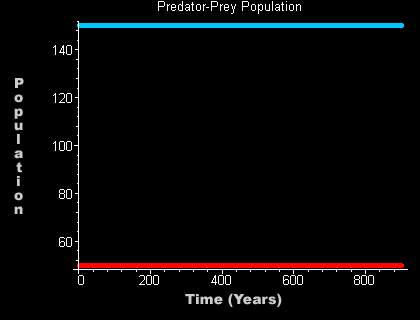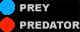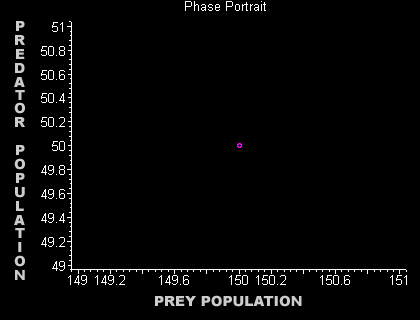### Equilibrium Points

##### Now, converting the variables back, x = Pn and y = Qn. The equilibrium point for the predators is a/b and the E.P. for the prey is d/c.  Plugging these points as the initial populations we get two constant growths as expected.##### When viewing the same initial population in the phase plot, we see the same constant point. Notice that the equilibrium point presented here is the center of the previous phase plot.